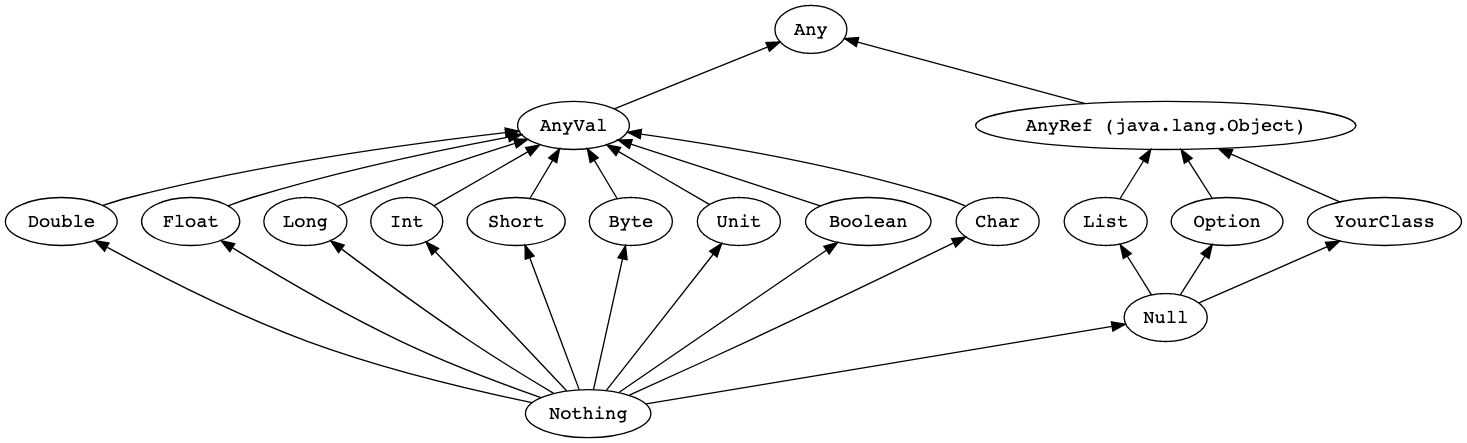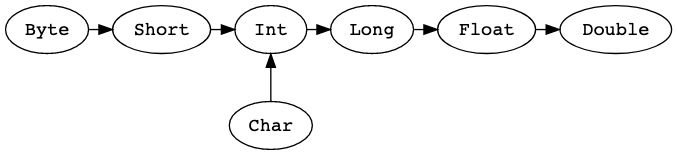# Scala教程之:面向对象的scala

## 面向对象的scala

• Unified Types
• Classes
• Traits

## Unified TypesAnyVal表示的是值类型，它有9个预定义的非空的值类型分别是：Double、Float、Long、Int、Short、Byte、Char、Unit和Boolean。

AnyRef表示的是引用类型。所有的非值的类型都是引用类型。并且所有的用户自定义类型都是AnyRef类型的子类。在Java环境中，AnyRef相当于Java里面的java.lang.Object。``````val x: Long = 987654321
val y: Float = x  // 9.8765434E8 (note that some precision is lost in this case)

val face: Char = '☺'
val number: Int = face  // 9786
``````

Nothing是所有类型的子类，它通常被用来表示非正常终止的信号，比如抛出异常，程序退出等等。

Null是所有引用类型的子类型，Null主要是用来跟JVM交互使用的，通常不需要在Scala中使用到它。

## Classes

Classes就是类，和java中的类相似，它里面可以包含方法、常量、变量、类型、对象、特质、类等。

``````class Family

val family = new Family
``````

new关键字是用来创建类的实例。在上面的例子中，Family没有定义构造器，所以默认带有一个无参的默认的构造器。

• 构造器

``````class Point(var x: Int, var y: Int) {

override def toString: String =
s"(\$x, \$y)"
}

val point1 = new Point(2, 3)
point1.x  // 2
println(point1)  // prints (2, 3)
``````

``````class Point(var x: Int = 0, var y: Int = 0)

val origin = new Point  // x and y are both set to 0
val point1 = new Point(1)
println(point1.x)  // prints 1

``````

``````class Point(val x: Int, val y: Int)
val point = new Point(1, 2)
point.x = 3  // <-- does not compile
``````

``````class Point(x: Int, y: Int)
val point = new Point(1, 2)
point.x  // <-- does not compile
``````
• 私有成员和Getter/Setter语法

Scala的成员默认是public的。如果想让其变成私有的，可以加上private修饰符，Scala的getter和setter语法和java不太一样，下面我们来举个例子：

``````class Point {

private var _x = 0
private var _y = 0
private val bound = 100

def x = _x
def x_= (newValue: Int): Unit = {
if (newValue < bound) _x = newValue else printWarning
}

def y = _y
def y_= (newValue: Int): Unit = {
if (newValue < bound) _y = newValue else printWarning
}

private def printWarning = println("WARNING: Out of bounds")
}

object Point {
def main(args: Array[String]): Unit = {
val point1 = new Point
point1.x = 99
point1.y = 101 // prints the warning
}
}
``````

## Traits

• 定义Traits

``````trait HelloWorld
``````

``````trait Iterator[A] {
def hasNext: Boolean
def next(): A
}
``````

• 使用Traits

``````trait Iterator[A] {
def hasNext: Boolean
def next(): A
}

class IntIterator(to: Int) extends Iterator[Int] {
private var current = 0
override def hasNext: Boolean = current < to
override def next(): Int =  {
if (hasNext) {
val t = current
current += 1
t
} else 0
}
}

val iterator = new IntIterator(10)
iterator.next()  // returns 0
iterator.next()  // returns 1
``````
• 子类型

``````import scala.collection.mutable.ArrayBuffer

trait Pet {
val name: String
}

class Cat(val name: String) extends Pet
class Dog(val name: String) extends Pet

val dog = new Dog("Harry")
val cat = new Cat("Sally")

val animals = ArrayBuffer.empty[Pet]
animals.append(dog)
animals.append(cat)
animals.foreach(pet => println(pet.name))  // Prints Harry Sally
``````